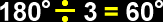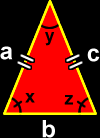# Types of Triangles

Definitions and formulas for triangles including right triangles, equilateral triangles, isosceles triangles, scalene triangles, obtuse triangles and acute triangles
Just scroll down or click on what you want and I'll scroll down for you!

 right triangle equilateral triangle isosceles triangle obtuse triangle acute triangle

 The right triangle:The right triangle has one 90 degree angle and two acute (< 90 degree) angles.  Since the sum of the angles of a triangle is always 180 degrees...y + z = 90 degrees The two sides of the triangle that are by the right angle are called the legs...  and the side opposite of the right angle is called the hypotenuse.The equilateral triangle:In the equilateral triangle, all the sides are the same length (congruent) and all the angles are the same size (congruent).  Since the sum of the angles of a triangle is always 180 degrees, we can figure out the measure of the angles of an equilateral triangle:The isosceles triangle:The isosceles triangle (I can NEVER remember how to spell isosceles) has two sides that are the same length (congruent) and two angles that are the same size (congruent).